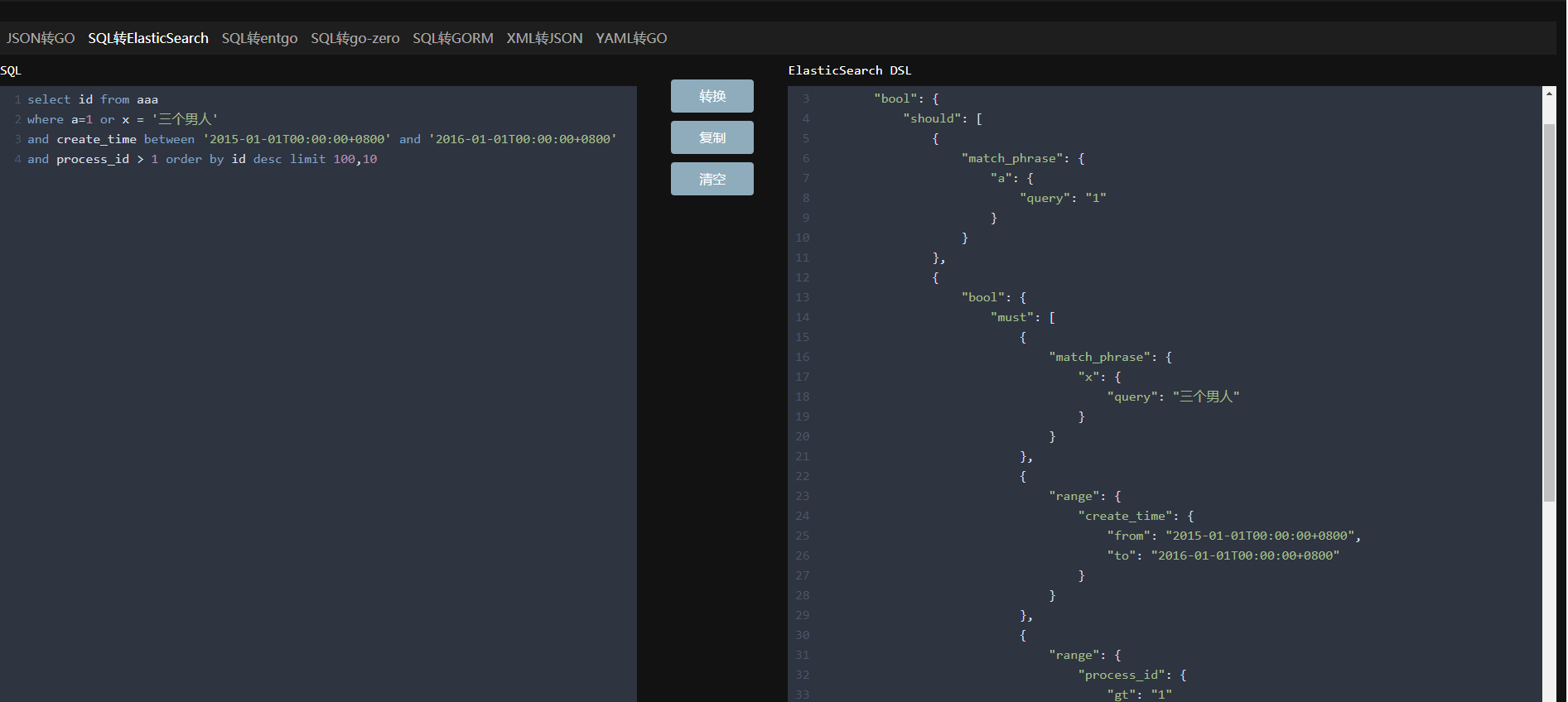# 分享 在线 SQL 转 ElasticSearch DSL

2825873215 · 2021年08月19日 · 304 次阅读

## 效果图## 当前支持

• [x] sql and expression
• [x] sql or expression
• [x] equal(=) support
• [x] not equal(!=) support
• [x] gt(>) support
• [x] gte(>=) support
• [x] lt(<) support
• [x] lte(<=) support
• [x] sql in (eg. id in (1,2,3) ) expression
• [x] sql not in (eg. id not in (1,2,3) ) expression
• [x] paren bool support (eg. where (a=1 or b=1) and (c=1 or d=1))
• [x] sql like expression (currently use match phrase, perhaps will change to wildcard in the future)
• [x] sql order by support
• [x] sql limit support
• [x] sql not like expression
• [x] field missing check
• [x] support aggregation like count(*), count(field), min(field), max(field), avg(field)
• [x] support aggregation like stats(field), extended_stats(field), percentiles(field) which are not standard sql function
• [ ] null check expression(is null/is not null)
• [ ] join expression
• [ ] having support